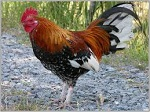# Tech Tips

1. Uncategorized
2. 84 view

## [Coq]Binarize with Proof Driven Development part1Contents

# Target

Try to develop function which binarize image with Proof Driven Development.

# Target

Try to develop function which binarize image with Proof Driven Development.

# Coq

Define binarization function with Coq.
```Require Import List.
Require Import Arith.

Fixpoint binarize (l : list nat)(th : nat) : list nat :=
match l with
| nil =&amp;amp;gt; nil
| x::xs =&amp;amp;gt; if leb th x then 255 :: (binarize xs th) else 0 :: (binarize xs th)
end.```
I think following things are needed for proofing.
• Same element number with the list and the list after applying function.
• The list which is applied the function has only 2 type value 0 or 255.
I’ll prove them from next.
Define binarization function with Coq.
```Require Import List.
Require Import Arith.

Fixpoint binarize (l : list nat)(th : nat) : list nat :=
match l with
| nil => nil
| x::xs => if leb th x then 255 :: (binarize xs th) else 0 :: (binarize xs th)
end.```
I think following things are needed for proofing.
• Same element number with the list and the list after applying function.
• The list which is applied the function has only 2 type value 0 or 255.
I’ll prove them from next.